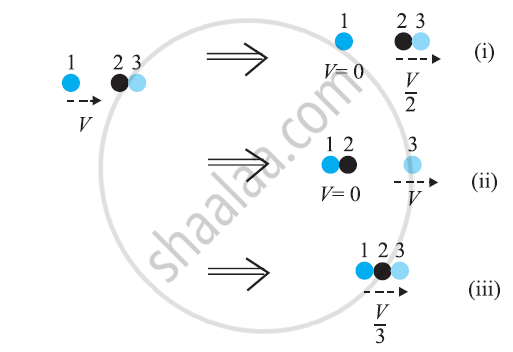CBSE (Science) Class 11CBSE
Share

# Two Identical Ball Bearings in Contact with Each Other and Resting on a Frictionless Table Are Hit Head-on by Another Ball Bearing of the Same Mass Moving Initially with a Speed V. If the Collision is Elastic, Which of the Following Figure is a Possible Result After Collision - CBSE (Science) Class 11 - Physics

ConceptConcept of Collisions

#### Question

Two identical ball bearings in contact with each other and resting on a frictionless table are hit head-on by another ball bearing of the same mass moving initially with a speed V. If the collision is elastic, which of the following figure is a possible result after collision?#### Solution 1

Let m be the mass of each ball bearing. Before collision, total K.E. of the system

=1/2mv2 + 0 =1/2 mv2

After collision, K.E. of the system is

Case I, E1 = 1/2 (2m) (v/2)2 = 1/4 mv2

Case II, E2 = 1/2 mv2

Case III, E3 = 1/2(3m) (v/3)2 = 1/6mv2

Thus, case II is the only possibility since K.E. is conserved in this case.

#### Solution 2

It can be observed that the total momentum before and after collision in each case is constant.

For an elastic collision, the total kinetic energy of a system remains conserved before and after collision.

For mass of each ball bearing m, we can write:

Total kinetic energy of the system before collision:

= (1/2)mV2 + (1/2)(2m) × 02

= (1/2)mV2

Case (i)
Total kinetic energy of the system after collision:

= (1/2) m × 0 + (1/2) (2m) (V/2)2

= (1/4)mV2

Hence, the kinetic energy of the system is not conserved in case (i).

Case (ii)
Total kinetic energy of the system after collision:

= (1/2)(2m) × 0 + (1/2)mV2

= (1/2) mV2

Hence, the kinetic energy of the system is conserved in case (ii).

Case (iii)

Total kinetic energy of the system after collision:

= (1/2)(3m)(V/3)2

= (1/6)mV2

Hence, the kinetic energy of the system is not conserved in case (iii).

Hence, Case II is the only possibility.

Is there an error in this question or solution?

#### APPEARS IN

NCERT Solution for Physics Textbook for Class 11 (2018 to Current)
Chapter 6: Work, Energy and Power
Q: 16 | Page no. 136
Solution Two Identical Ball Bearings in Contact with Each Other and Resting on a Frictionless Table Are Hit Head-on by Another Ball Bearing of the Same Mass Moving Initially with a Speed V. If the Collision is Elastic, Which of the Following Figure is a Possible Result After Collision Concept: Concept of Collisions.
S